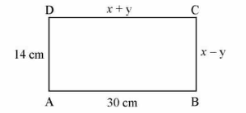# Class 10 – Pair of Linear Equations in Two Variables – Previous Years Questions

Pair of Linear Equations in Two Variables – Previous Years Questions

1. The pair of linear equations y = 0 and y = -6 has
1. a unique solution
2. no solution
3. infinitely many solution
4. only solution (0,0) [CBSE 2020] [1 Mark]
2. Solve the following pair of linear equations:
3x – 5y = 4
2y + 7 = 9x [CBSE 2019] [2 Marks]
3. Solve the following pair of linear equations:
3x + 4y = 10
2x – 2y = 2 [CBSE 2019] [2 Marks]
4. In figure ABCD is a rectangle. Find the value of X and Y.[CBSE 2018] [2 Marks]
5. Solve the pair of equations:
2/x + 3/y = 11, 5/x – 4/y = -7
Hence, find the value of 5x – 3y. [CBSE 2020] [3 Marks]
6. Taxi charges in a city consist of fixed charges and the remaining charges depend upon the distance travelled. For a journey of 10 km, the charge paid is Rs 75 and for a journey of 15 km, the charge paid is Rs 110. Find the fixed charge and charges per km. Hence, find the charge of covering a distance of 35 km. [CBSE 2020] [3 Marks]
7. Draw the graph of the equations x – y = 1 and 3x + 2y – 12 = 0. Using this graph, find the values of x and y which satisfy booth the equations. [CBSE 2019] [3 Marks]
8. Two numbers are in the ratio of 5:6. If 7 is subtracted from each of the numbers, the ratio becomes 4:5. Find the numbers. [CBSE 2019] [3 Marks]